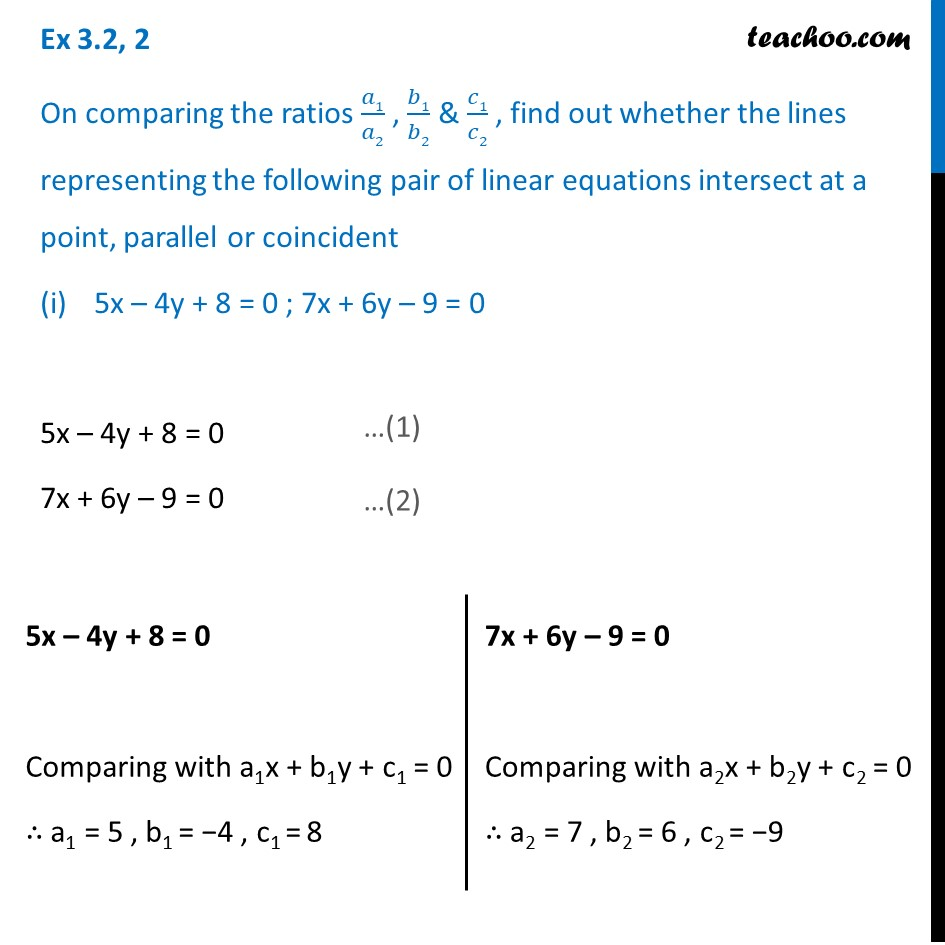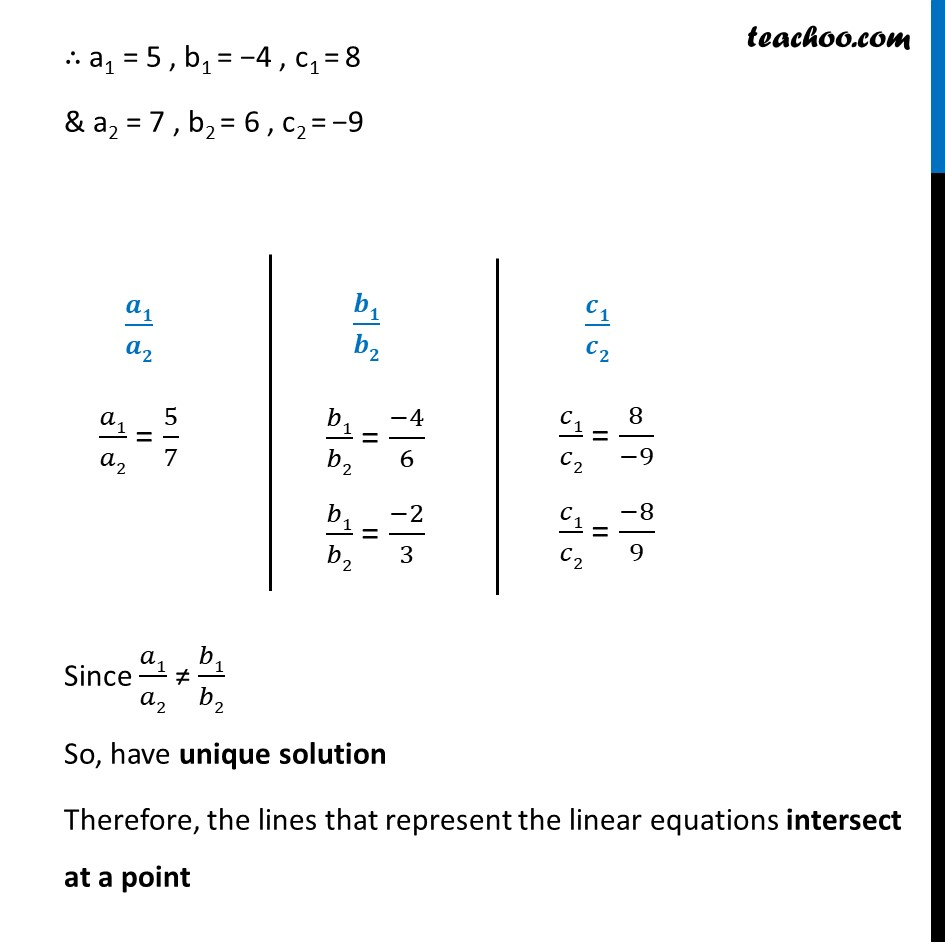1. Chapter 3 Class 10 Pair of Linear Equations in Two Variables (Term 1)
2. Concept wise
3. Finding ratios (Consistency)

Transcript

Ex 3.2, 2 On comparing the ratios 𝑎1/𝑎2 , 𝑏1/𝑏2 & 𝑐1/𝑐2 , find out whether the lines representing the following pair of linear equations intersect at a point, parallel or coincident 5x – 4y + 8 = 0 ; 7x + 6y – 9 = 0 5x – 4y + 8 = 0 7x + 6y – 9 = 0 5x – 4y + 8 = 0 Comparing with a1x + b1y + c1 = 0 ∴ a1 = 5 , b1 = −4 , c1 = 8 7x + 6y – 9 = 0 Comparing with a2x + b2y + c2 = 0 ∴ a2 = 7 , b2 = 6 , c2 = −9 ∴ a1 = 5 , b1 = −4 , c1 = 8 & a2 = 7 , b2 = 6 , c2 = −9 𝒂𝟏/𝒂𝟐 𝑎1/𝑎2 = 5/7 𝒃𝟏/𝒃𝟐 𝑏1/𝑏2 = (−4)/6 𝑏1/𝑏2 = (−2)/3 𝒄𝟏/𝒄𝟐 𝑐1/𝑐2 = 8/(−9) 𝑐1/𝑐2 = (−8)/9 Since 𝑎1/𝑎2 ≠ 𝑏1/𝑏2 So, have unique solution Therefore, the lines that represent the linear equations intersect at a point